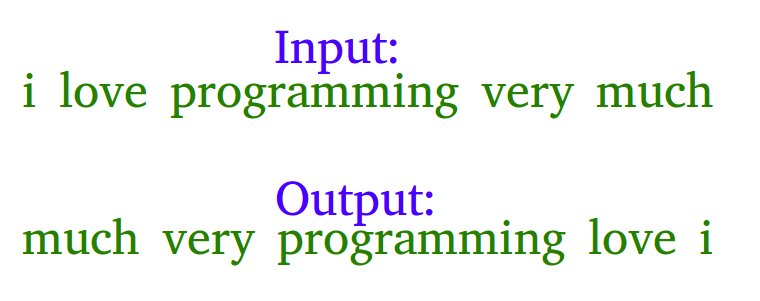GeeksforGeeks App
Open AppBrowser
Continue

# C# Program To Reverse Words In A Given String

Example: Let the input string be “i like this program very much”. The function should change the string to “much very program this like i”Examples

Input: s = “geeks quiz practice code”
Output: s = “code practice quiz geeks”

Input: s = “getting good at coding needs a lot of practice”
Output: s = “practice of lot a needs coding at good getting”

Algorithm:

• Initially, reverse the individual words of the given string one by one, for the above example, after reversing individual words the string should be “i ekil siht margorp yrev hcum”.
• Reverse the whole string from start to end to get the desired output “much very program this like i” in the above example.

Below is the implementation of the above approach:

## C#

 `// C# program to reverse``// a String``using` `System;``class` `GFG{` `// Reverse the letters``// of the word``static` `void` `reverse(``char` `[]str,``                    ``int` `start, ``int` `end)``{``  ` `  ``// Temporary variable to``  ``// store character``  ``char` `temp;``  ` `  ``while` `(start <= end)``  ``{``    ` `    ``// Swapping the first and``    ``// last character``    ``temp = str[start];``    ``str[start] = str[end];``    ``str[end] = temp;``    ``start++;``    ``end--;``  ``}``}``  ` `// Function to reverse words``static` `char``[] reverseWords(``char` `[]s)``{ ``  ``// Reversing individual words as``  ``// explained in the first step` `  ``int` `start = 0;``  ``for` `(``int` `end = 0;``           ``end < s.Length; end++)``  ``{   ``    ``// If we see a space, we reverse``    ``// the previous word (word between``    ``// the indexes start and end - 1``    ``// i.e., s[start..end-1]``    ``if` `(s[end] == ``' '``)``    ``{``      ``reverse(s, start, end);``      ``start = end + 1;``    ``}``  ``}` `  ``// Reverse the last word``  ``reverse(s, start, s.Length - 1);` `  ``// Reverse the entire String``  ``reverse(s, 0, s.Length - 1);``  ``return` `s;``}` `// Driver Code``public` `static` `void` `Main(String[] args)``{``  ``String s =``  ``"i like this program very much "``;``  ``char` `[]p = reverseWords(s.ToCharArray());``  ``Console.Write(p);``}``}``// This code is contributed by jana_sayantan`

Output:

`much very program this like i`

Another Approach:

we can do the above task by splitting and saving the string in a reverse manner.

Below is the implementation of the above approach:

## C#

 `// C# program to reverse a string``using` `System;``public` `class` `ReverseWords``{``    ``public` `static` `void` `Main()``    ``{``        ``string``[] s =``        ``"i like this program very much"``.Split(``' '``);``        ``string` `ans = ``""``;``        ``for` `(``int` `i = s.Length - 1;``                 ``i >= 0; i--)``        ``{``            ``ans += s[i] + ``" "``;``        ``}``        ``Console.Write(``        ``"Reversed String:"``);``        ``Console.Write(ans.Substring(0,``                      ``ans.Length - 1));``    ``}``}`

Output:

```Reversed String:
much very program this like i```

Time Complexity: O(n)

Auxiliary Space: O(n)

Without using any extra space:
The above task can also be accomplished by splitting and directly swapping the string starting from the middle. As direct swapping is involved, less space is consumed too.

Below is the implementation of the above approach:

## C#

 `// C# code to reverse a string``using` `System;``class` `GFG{``    ` `// Reverse the string``public` `static` `String[] RevString(String[] s,``                                 ``int` `l)``{   ``    ``// Check if number of words is even``    ``if` `(l % 2 == 0)``    ``{       ``        ``// Find the middle word``        ``int` `j = l / 2;``        ` `        ``// Starting from the middle``        ``// start swapping words at``        ``// jth position and l-1-j position``        ``while` `(j <= l - 1)``        ``{``            ``String temp;``            ``temp = s[l - j - 1];``            ``s[l - j - 1] = s[j];``            ``s[j] = temp;``            ``j += 1;``        ``}``    ``}``    ` `    ``// Check if number of words is odd``    ``else``    ``{       ``        ``// Find the middle word``        ``int` `j = (l / 2) + 1;``        ` `        ``// Starting from the middle start``        ``// swapping the words at jth``        ``// position and l-1-j position``        ``while` `(j <= l - 1)``        ``{``            ``String temp;``            ``temp = s[l - j - 1];``            ``s[l - j - 1] = s[j];``            ``s[j] = temp;``            ``j += 1;``        ``}``    ``}``    ` `    ``// Return the reversed sentence``    ``return` `s;``}` `// Driver Code``public` `static` `void` `Main(String[] args)``{``    ``String s = ``"getting good at coding "` `+``               ``"needs a lot of practice"``;``    ``String[] words = s.Split(``"\s"``);   ``    ``words = RevString(words, words.Length);   ``    ``s = String.Join(``" "``, words);   ``    ``Console.WriteLine(s);``}``}``// This code is contributed by shivanisinghss2110`

Output:

`practice of lot a needs coding at good getting`

Time Complexity: O(n)

Auxiliary Space: O(1)

Please refer complete article on Reverse words in a given string for more details!

My Personal Notes arrow_drop_up